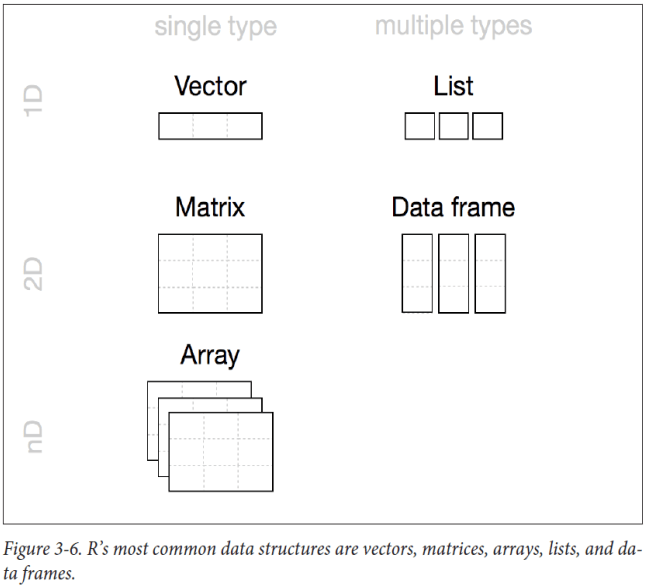# R Tutorial 4: Data Structures

Before we start off the chapter on data structure, this might be a little disorientating for people who learnt any other programming language, but R starts indexing from 1 instead of 0.

## Vectors

So what’s a vector? Vector is a type of R Object, that allows you to store a sequence of data elements of the same type. Read more at http://www.r-tutor.com/r-introduction/vector

e.g c(2,3,5) is a vector, where 2,3,5 are numerical elements of the vector.
c(“a”,“w”,“e”,“s”,“o”,“m”,“e”) is also a vector, where the letters are character elements.
c(True,False,False) is called a boolean vector, which can be useful for data analysis as well
Note that characters have to be quoted with “ or ‘, whereas numbers do not.

Let’s start by creating empty vectors of length 5 for numbers and characters

```n<-numeric(5)
n
##  0 0 0 0 0```
```m<-character(5)
m
##  "" "" "" "" ""```

What if I want to create a string of pre-filled numbers? You saw it on top, you can use the c() function, and put the values into the brackets, where R will automatically identify the data type of the vector.

```n<-c(1,2,3,4,5)
n
##  1 2 3 4 5```
```m<-c(6:10)
#this mean creating a number vector starting from 6 to 10, which is a handy way of creating a long sequence!
m
##   6  7  8  9 10```
```x <- n+m
#one interesting thing about R is that you can perform additions of vectors of the same length
just by doing the above, rather than accessing each elements of the vectors!
x
##   7  9 11 13 15```
```#Some useful functions in managing vectors will be finding the length of the vector
length(x)
##  5```
```#You can access the value at nth position of the vector by the following x[n]
x
##  9```

## List

List is similar to vectors, except it can take in any data type within itself, including vectors themselves

```newcustomer <- list("Benny",24,2,"M")
newcustomer
```
```## []
##  "Benny"
##
## []
##  24
##
## []
##  2
##
## []
##  "M"
```

## Matrix

Matrices are vectors that are in a table form – with rows and columns.
You can create a matrix by feeding a vector into the matrix() function

```a<- c(1,2,3,4,5,6)
#matrix function fills the matrix col by col by default
m <- matrix(a,nrow=2)
m
##      [,1] [,2] [,3]
## [1,]    1    3    5
## [2,]    2    4    6
```
```#if you want it to fill it by row, just include an additional arguments
n <- matrix(a,nrow=2,byrow = TRUE)
n
##      [,1] [,2] [,3]
## [1,]    1    2    3
## [2,]    4    5    6
```
```#if you want to access the value in the xth row and yth column of matrix n, you can do it via n[x,y]
n[2,3]
##  6```
```n[2,]
##  4 5 6```
```n[,3]
##  3 6```

## Dataframes

Dataframes are the most common data structure you will handle during the process of learning R. Dataframes group vectors of different data types into a 2D table. You can create a dataframe with the function data.frame()

```Name <- c("John","Thomas","Alice")
Age <- c(20,25,35)
Experience <- c(2,3,8)
People <- data.frame(Name,Age,Experience)
#now this dataframe has three vectors that contain character and numeric values.
People
```
```##     Name Age Experience
## 1   John  20          2
## 2 Thomas  25          3
## 3  Alice  35          8
```

So what if you want to add columns or rows to this data frame? We use two functions, cbind() and rbind()

```Name <- as.character(c("John","Thomas","Alice"))
Age <- c(20,25,35)
Experience <- c(2,3,8)
People <- data.frame(Name,Age,Experience)
#adding another column of data for gender.
#now you realise you have to use a vector because it support 1 data type only.
Sex <- c("M","M","F")
People <- cbind(People,Sex)
People
```
```##     Name Age Experience Sex
## 1   John  20          2   M
## 2 Thomas  25          3   M
## 3  Alice  35          8   F
```
```#adding another row of data for a new individual
newcustomer <- data.frame(Name = "Betty", Age=24, Experience = 2, Sex = "F")
UpdatedPeople <- rbind(People,newcustomer)
UpdatedPeople
```
```##     Name Age Experience Sex
## 1   John  20          2   M
## 2 Thomas  25          3   M
## 3  Alice  35          8   F
## 4  Betty  24          2   F
```

## Classes

We have been discussing different types of data in vectors and dataframes, but so far we only covered two types of classes (or data types) in general, which are numeric and character. There are also logical and factor.

• Numeric (Two types of numeric classes, “Double” is for decimals, and Integer is for … integer)
• Character (Strings of characters are also considered characters)
• Logical (True or False)
• Factor (Categorical Information)
```#numerical data structures are automatically assigned to the numerical class
age <- c(15,17,18)

#same for logical vectors, also T and F are shorthands of True and False
married <- c(T,F,F)
#factors need to be assigned separately, else it will be treated as characters.
It is important to change data into factor for R to process it for linear regression which we will cover later.
sex <- factor(c("male","female"))

class(age)
##  "numeric"

class(sex)
##  "factor"

class(married)
##  "logical"```

## Other Data Structures

There are also other data structure that are useful for different purposes, such as list when you need to store a sequence of information of different types, or an array for a multidimensional table. For constraint of time, these are not covered as they are usually used for scientific calculations.

The features of different type of data structures are nicely summarised in the diagrams below.Next: Tutorial 5 Basic Programming (Continued)